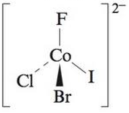# Which of the following molecules exhibit(s) optical isomerism? a . cis -Pt(NH 3 ) 2 Cl 2 b . trans -Ni(en) 2 Br 2 (en is ethylenediamine) c. cis -Ni(en) 2 Br 2 (en is ethylenediamine) d .### Chemistry: An Atoms First Approach

2nd Edition
Steven S. Zumdahl + 1 other
Publisher: Cengage Learning
ISBN: 9781305079243

#### Solutions

Chapter
Section### Chemistry: An Atoms First Approach

2nd Edition
Steven S. Zumdahl + 1 other
Publisher: Cengage Learning
ISBN: 9781305079243
Chapter 20, Problem 84CWP
Textbook Problem
3 views

## Which of the following molecules exhibit(s) optical isomerism?a. cis-Pt(NH3)2Cl2b. trans-Ni(en)2Br2 (en is ethylenediamine)c. cis-Ni(en)2Br2 (en is ethylenediamine)d.(a)

Interpretation Introduction

Interpretation: The molecules that exhibit optical isomerism are to be determined.

Concept introduction: If the two molecules have same number of atoms and their bonding is also same but their mirror images are non super imposable on each other, then the molecules exhibits optical isomerism.

Optical isomerism is a type of stereoisomerism. Optical isomers are able to rotate the plane polarized light.

To determine: If Cis- Pt(NH3)Cl2 exhibits optical isomerism or not.

### Explanation of Solution

Explanation

Figure 1

The given molecule is cis- Pt(

(b)

Interpretation Introduction

Interpretation: The molecules that exhibit optical isomerism are to be determined.

Concept introduction: If the two molecules have same number of atoms and their bonding is also same but their mirror images are non super imposable on each other, then the molecules exhibits optical isomerism.

Optical isomerism is a type of stereoisomerism. Optical isomers are able to rotate the plane polarized light.

To determine: If trans- Ni(en)2Br2 do not exhibits optical isomerism or not.

(c)

Interpretation Introduction

Interpretation: The molecules that exhibit optical isomerism are to be determined.

Concept introduction: If the two molecules have same number of atoms and their bonding is also same but their mirror images are non super imposable on each other, then the molecules exhibits optical isomerism.

Optical isomerism is a type of stereoisomerism. Optical isomers are able to rotate the plane polarized light.

To determine: If Cis- Ni(en)2Br2 exhibits optical isomerism or not.

(d)

Interpretation Introduction

Interpretation: The molecules that exhibit optical isomerism are to be determined.

Concept introduction: If the two molecules have same number of atoms and their bonding is also same but their mirror images are non super imposable on each other, then the molecules exhibits optical isomerism.

Optical isomerism is a type of stereoisomerism. Optical isomers are able to rotate the plane polarized light.

To determine: If [Co(Br)(Cl)(F)(I)]2 exhibits optical isomerism or not.

### Still sussing out bartleby?

Check out a sample textbook solution.

See a sample solution

#### The Solution to Your Study Problems

Bartleby provides explanations to thousands of textbook problems written by our experts, many with advanced degrees!

Get Started

Find more solutions based on key concepts
Calorie deficit is no longer the primary strategy for weight management.

Nutrition: Concepts and Controversies - Standalone book (MindTap Course List)

Consider a graphical representation (Fig. 15.3) of simple harmonic motion as described mathematically in Equati...

Physics for Scientists and Engineers, Technology Update (No access codes included)

How are diatoms similar to and different from dinoflagellates?

Oceanography: An Invitation To Marine Science, Loose-leaf Versin